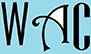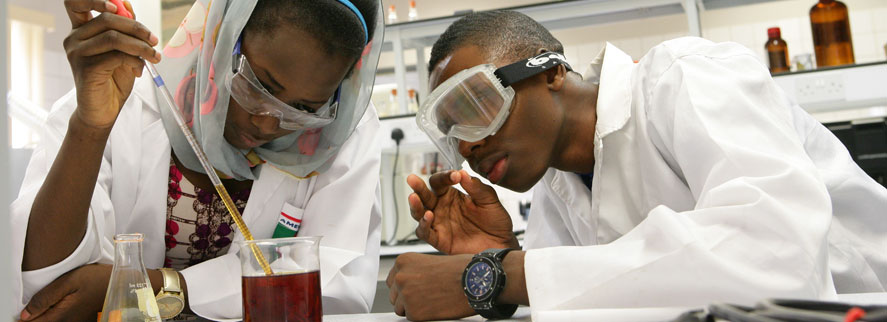## Algebra Question for Title 1 Schools: Percent of What Number Question?

14) 35% of what number is 70?

35% = 35/100 = 0.35

of = x (multiply)

0.35x = 70

you have to make “x” subject of formula by moving “0.35” to the other side. When you go to the other side, addition becomes subtraction, multiplication becomes division, and vice versa.

x = 70/0.35 (0.35 was a multiplication at the other end)

x = 200